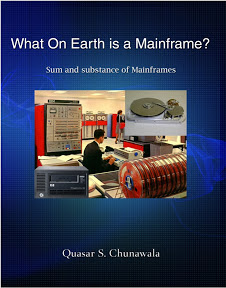Mainframes 360
The one stop destination for System Z professionals

## Saturday, September 26, 2009

### Arithmetic operators

 Q. What are the Arithmetic Operators in Cobol? Cobol has five standard arithmetic verbs, ADD, SUBTRACT, MULTIPLY, DIVIDE and COMPUTE. Arithmetic statements can be written in various different forms. Look at the examples below. The ADD verb adds up the first number TO the second. If you write ADD X TO Y, it means Y + X. If you write ADD X Y TO Z, it means (i) first add X to Z (ii)second add Y to Z. The result of addition is always stored in the second. If an optional GIVING keyword is specified on the ADD statement, the result of the addition is assigned to a third variable. The SUBTRACT verb subtracts the first number FROM the second. If you write SUBTRACT Y FROM X, it means X – Y. If you write SUBTRACT X Y FROM W, it means (i) first subtract X from W (ii)second subtract Y from W. The difference is stored in the second. The MULTIPLY verb computes the product of the first times the second. If you write MULTIPLY X BY Y, X is multiplied with Y, and the product is stored in Y.  When DIVIDE 10 BY 05, the result is 2. If you DIVIDE 3 INTO 6, the result is 2. On dividing 10 BY 3, the remainder is 1. The COMPUTE verb in Cobol is for writing arithmetic expressions. For example, to calculate the area of a circle, you may write COMPUTE AREA = 3.14159 * (RADIUS ** 2). The COMPUTE Statement has the general form COMPUTE result = arithmetic-expression. On the Left-Hand side(LHS), you write the variable, which shall store the result of computation. On the RHS, you write the arithmetic expression whose value needs to be evaluated. The table below contains examples of how arithmetic operators in Cobol are used.Sample Program Listing A quadratic equation(highest degree=2) has the general form ax2 + bx + c = 0. The formula for calculating the roots of a quadratic equation is. A Cobol program has to be designed and coded to compute the quadratic roots, with precision upto four digits after the decimal point. Program SourceTo many people who are thrown to work at a mainframe computer on their first job, they feel lost. Mainframe people seem to speak a completely different language and that doesn't make life easy. What's more, the books and manuals are incredibly hard to comprehend.

"What on earth is a Mainframe?" is an absolute beginner's guide to mainframe computers. We'll introduce you to the hardware and peripherals. We'll talk about the operating system, the software installed on a mainframe. We'll also talk about the different people who work on a mainframe. In a nutshell, we'll de-mystify the mainframe.

Readers based in India, can buy the e-book for Rs. 50 only or the print book. International readers based in the US and other countries can click here to purchase the e-book.